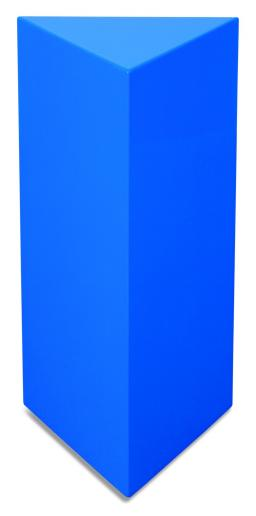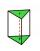# Right triangular prism

We have a cuboid with a base and dimensions of 12 cm and 5 cm and a height of 4 cm. The tablecloth is cut into two identical triangular prisms with right triangular bases. We painted the surface of the created prisms with color. Calculate the surface area of one of these two triangular prisms.

S =  180 cm2

### Step-by-step explanation:Did you find an error or inaccuracy? Feel free to write us. Thank you!Tips to related online calculators
Pythagorean theorem is the base for the right triangle calculator.

#### You need to know the following knowledge to solve this word math problem:

We encourage you to watch this tutorial video on this math problem:

## Related math problems and questions:

• Rhombus baseCalculate the volume and surface area of prisms whose base is a rhombus with diagonals u1 = 12 cm and u2 = 15 cm. Prism height is twice the base edge length.
• Triangular prism - regularThe regular triangular prism is 7 cm high. Its base is an equilateral triangle whose height is 3 cm. Calculate the surface and volume of this prism.
• Four sided prismCalculate the volume and surface area of a regular quadrangular prism whose height is 28.6cm and the body diagonal forms a 50-degree angle with the base plane.
• Triangular prismThe base of the perpendicular triangular prism is a rectangular triangle with a hypotenuse of 10 cm and one leg of 8 cm. The prism height is 75% of the perimeter of the base. Calculate the volume and surface of the prism.
• Body diagonalCalculate the volume of a cuboid whose body diagonal u is equal to 6.1 cm. Rectangular base has dimensions of 3.2 cm and 2.4 cm
• Total areaCalculate the total area (surface and bases) of a prism whose base is a rhombus which diagonals of 12cm and 18cm and prism height are 10 cm.
• Height of the cuboidCuboid with a rectangular base, measuring 3 cm and 4 cm diagonal has a body 13 centimeters long. What is the height of the cuboid?
• PrismThe base of a perpendicular triangular prism is a right triangle with legs 4.5 cm and 6 cm long. What is the surface of the prism, if its volume is 54 cubic centimeters?
• Triangular prismThe base perpendicular triangular prism is a right triangle whose hypotenuse measures 5 cm and one cathetus 2 cm. Height of the prism is equal to 7/9 of the perimeter of the base. Calculate the surface area of prism.
• Triangular prismThe triangular prism has a base in the shape of a right triangle, the legs of which is 9 cm and 40 cm long. The height of the prism is 20 cm. What is its volume cm3? And the surface cm2?
• Triangular prismCalculate the surface area and volume of a triangular prism, base right triangle if a = 3 cm, b = 4 cm, c = 5 cm, and height of prism h=12 cm.
• Prism - boxThe base of prism is a rectangle with a side of 7.5 cm and 12.5 cm diagonal. The volume of the prism is V = 0.9 dm3. Calculate the surface of the prism.
• Regular square prismThe volume of a regular square prism is 192 cm3. The size of its base edge and the body height is 1: 3. Calculate the surface of the prism.
• Cuboid easyThe cuboid has the dimensions a = 12 cm, b = 9 cm, c = 36 cm. Calculate the length of the body diagonal of the cuboid.
• Base of prismThe base of the perpendicular prism is a rectangular triangle whose legs length are at a 3: 4 ratio. The height of the prism is 2cm smaller than the larger base leg. Determine the volume of the prism if its surface is 468 cm2.Calculate the volume and surface area of a regular quadrangular prism 35 cm high and the base diagonal of 22 cm.The base of the perpendicular triangular prism is a right triangle with a leg length of 5 cm. The content area of the largest sidewall of its surface is 130 cm², and the height of the body is 10 cm. Calculate its volume.